Скачать презентацию Year 7 Maths Homework Summer term Each

07004422de38f32a0742db592edd89a8.ppt

• Количество слайдов: 13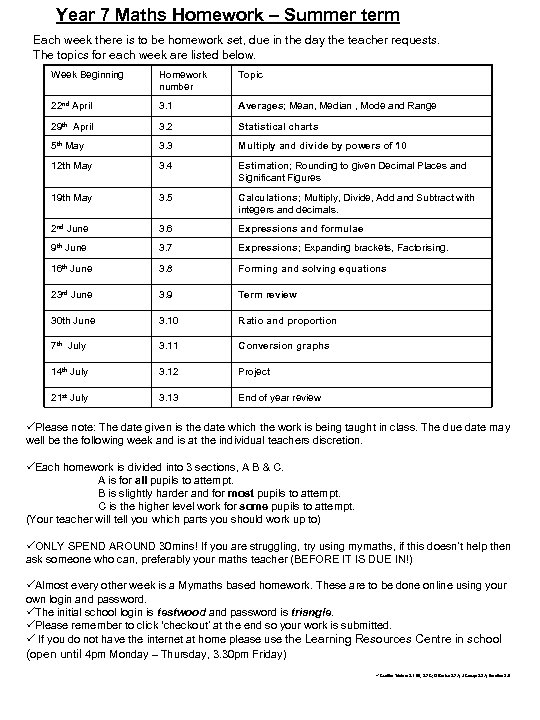Year 7 Maths Homework – Summer term Each week there is to be homework set, due in the day the teacher requests. The topics for each week are listed below. Week Beginning Homework number Topic 22 nd April 3. 1 Averages; Mean, Median , Mode and Range 29 th April 3. 2 Statistical charts 5 th May 3. 3 Multiply and divide by powers of 10 12 th May 3. 4 Estimation; Rounding to given Decimal Places and Significant Figures 19 th May 3. 5 Calculations; Multiply, Divide, Add and Subtract with integers and decimals. 2 nd June 3. 6 Expressions and formulae 9 th June 3. 7 Expressions; Expanding brackets, Factorising. 16 th June 3. 8 Forming and solving equations 23 rd June 3. 9 Term review 30 th June 3. 10 Ratio and proportion 7 th July 3. 11 Conversion graphs 14 th July 3. 12 Project 21 st July 3. 13 End of year review üPlease note: The date given is the date which the work is being taught in class. The due date may well be the following week and is at the individual teachers discretion. üEach homework is divided into 3 sections, A B & C. A is for all pupils to attempt. B is slightly harder and for most pupils to attempt. C is the higher level work for some pupils to attempt. (Your teacher will tell you which parts you should work up to) üONLY SPEND AROUND 30 mins! If you are struggling, try using mymaths, if this doesn’t help then ask someone who can, preferably your maths teacher (BEFORE IT IS DUE IN!) üAlmost every other week is a Mymaths based homework. These are to be done online using your own login and password. üThe initial school login is testwood and password is triangle. üPlease remember to click ‘checkout’ at the end so your work is submitted. ü If you do not have the internet at home please use the Learning Resources Centre in school (open until 4 pm Monday – Thursday, 3. 30 pm Friday) üCredits: Tristan: 3. 1 B 4, 3. 7 C; D Burke: 3. 7 A; J Casey: 3. 3 A; Emaths: 3. 8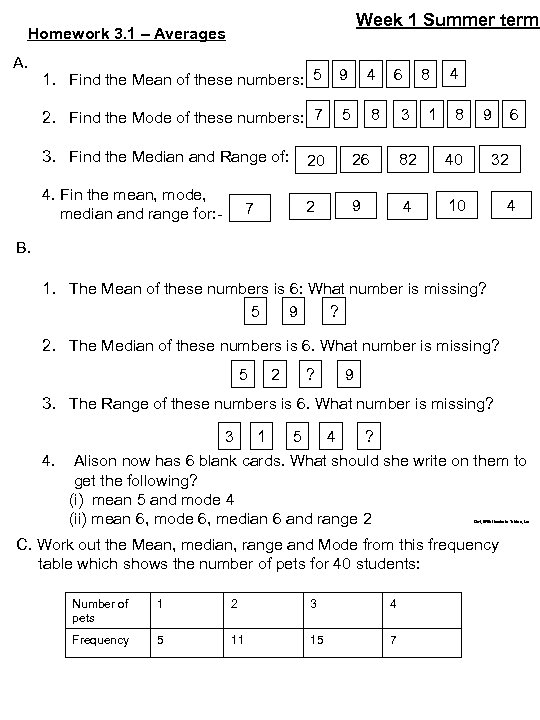Week 1 Summer term Homework 3. 1 – Averages A. 1. Find the Mean of these numbers: 5 9 4 2. Find the Mode of these numbers: 7 5 6 8 8 3 1 4 8 3. Find the Median and Range of: 20 26 82 40 4. Fin the mean, mode, median and range for: - 2 9 4 10 7 6 9 32 4 B. 1. The Mean of these numbers is 6: What number is missing? 5 9 ? 2. The Median of these numbers is 6. What number is missing? 5 2 ? 9 3. The Range of these numbers is 6. What number is missing? 3 1 5 4 ? 4. Alison now has 6 blank cards. What should she write on them to get the following? (i) mean 5 and mode 4 (ii) mean 6, mode 6, median 6 and range 2 Qn 4, With thanks to Tristan, tes C. Work out the Mean, median, range and Mode from this frequency table which shows the number of pets for 40 students: Number of pets 1 2 3 4 Frequency 5 11 15 7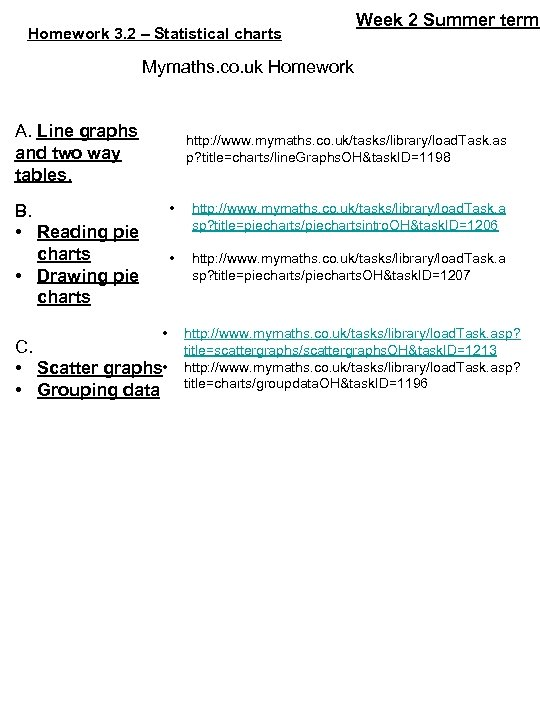Homework 3. 2 – Statistical charts Week 2 Summer term Mymaths. co. uk Homework A. Line graphs and two way tables. http: //www. mymaths. co. uk/tasks/library/load. Task. as p? title=charts/line. Graphs. OH&task. ID=1198 • • C. • Scatter graphs • • Grouping data http: //www. mymaths. co. uk/tasks/library/load. Task. a sp? title=piecharts/piechartsintro. OH&task. ID=1206 • B. • Reading pie charts • Drawing pie charts http: //www. mymaths. co. uk/tasks/library/load. Task. a sp? title=piecharts/piecharts. OH&task. ID=1207 http: //www. mymaths. co. uk/tasks/library/load. Task. asp? title=scattergraphs/scattergraphs. OH&task. ID=1213 http: //www. mymaths. co. uk/tasks/library/load. Task. asp? title=charts/groupdata. OH&task. ID=1196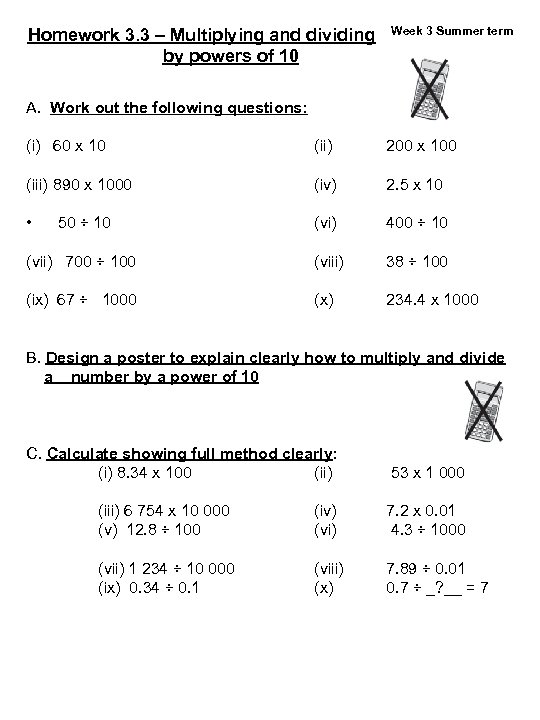Homework 3. 3 – Multiplying and dividing by powers of 10 A. Work out the following questions: (i) 60 x 10 (ii) (iii) 890 x 1000 (iv) • 50 ÷ 10 (vi) Week 3 Summer term 200 x 100 2. 5 x 10 400 ÷ 10 (vii) 700 ÷ 100 (viii) 38 ÷ 100 (ix) 67 ÷ 1000 (x) 234. 4 x 1000 B. Design a poster to explain clearly how to multiply and divide a number by a power of 10 C. Calculate showing full method clearly: (i) 8. 34 x 100 (ii) 53 x 1 000 (iii) 6 754 x 10 000 (v) 12. 8 ÷ 100 (iv) (vi) 7. 2 x 0. 01 4. 3 ÷ 1000 (vii) 1 234 ÷ 10 000 (ix) 0. 34 ÷ 0. 1 (viii) (x) 7. 89 ÷ 0. 01 0. 7 ÷ _? __ = 7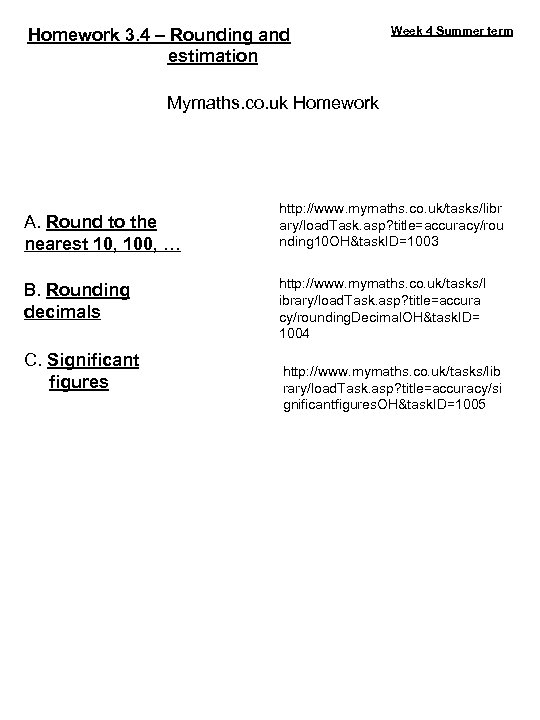Homework 3. 4 – Rounding and estimation Week 4 Summer term Mymaths. co. uk Homework A. Round to the nearest 10, 100, … B. Rounding decimals C. Significant figures http: //www. mymaths. co. uk/tasks/libr ary/load. Task. asp? title=accuracy/rou nding 10 OH&task. ID=1003 http: //www. mymaths. co. uk/tasks/l ibrary/load. Task. asp? title=accura cy/rounding. Decimal. OH&task. ID= 1004 http: //www. mymaths. co. uk/tasks/lib rary/load. Task. asp? title=accuracy/si gnificantfigures. OH&task. ID=1005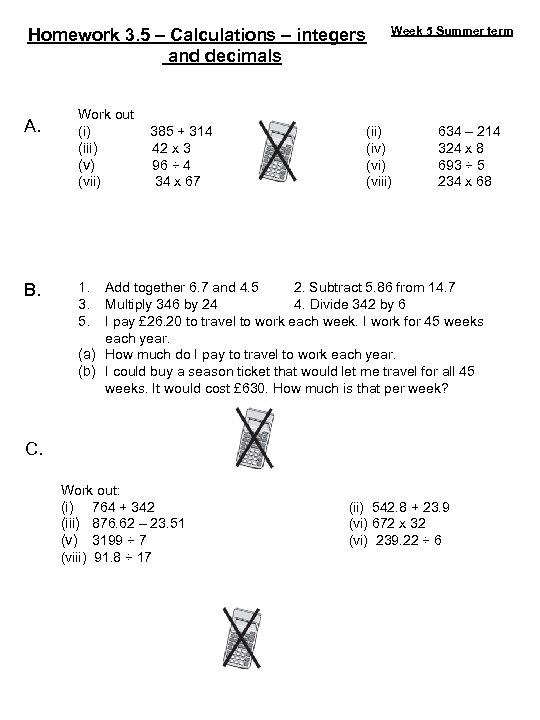Homework 3. 5 – Calculations – integers and decimals A. B. Work out (i) 385 + 314 (iii) 42 x 3 (v) 96 ÷ 4 (vii) 34 x 67 Week 5 Summer term (ii) (iv) (viii) 634 – 214 324 x 8 693 ÷ 5 234 x 68 1. Add together 6. 7 and 4. 5 2. Subtract 5. 86 from 14. 7 3. Multiply 346 by 24 4. Divide 342 by 6 5. I pay £ 26. 20 to travel to work each week. I work for 45 weeks each year. (a) How much do I pay to travel to work each year. (b) I could buy a season ticket that would let me travel for all 45 weeks. It would cost £ 630. How much is that per week? C. Work out: (i) 764 + 342 (iii) 876. 62 – 23. 51 (v) 3199 ÷ 7 (viii) 91. 8 ÷ 17 (ii) 542. 8 + 23. 9 (vi) 672 x 32 (vi) 239. 22 ÷ 6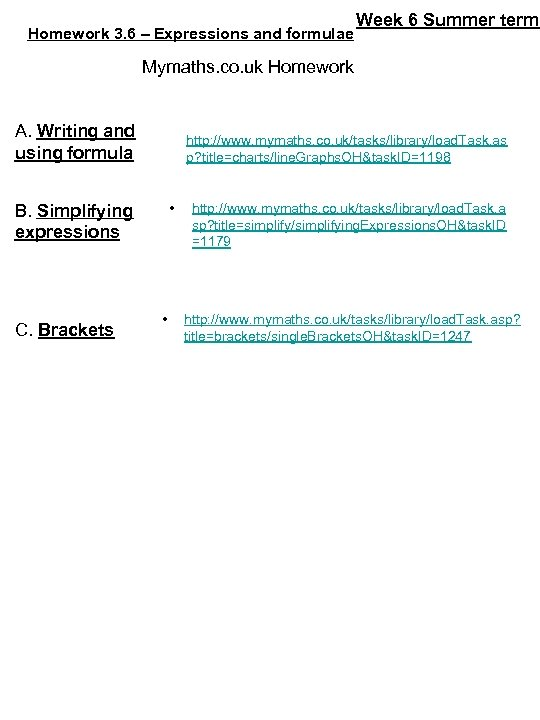Homework 3. 6 – Expressions and formulae Week 6 Summer term Mymaths. co. uk Homework A. Writing and using formula http: //www. mymaths. co. uk/tasks/library/load. Task. as p? title=charts/line. Graphs. OH&task. ID=1198 • B. Simplifying expressions C. Brackets • http: //www. mymaths. co. uk/tasks/library/load. Task. a sp? title=simplify/simplifying. Expressions. OH&task. ID =1179 http: //www. mymaths. co. uk/tasks/library/load. Task. asp? title=brackets/single. Brackets. OH&task. ID=1247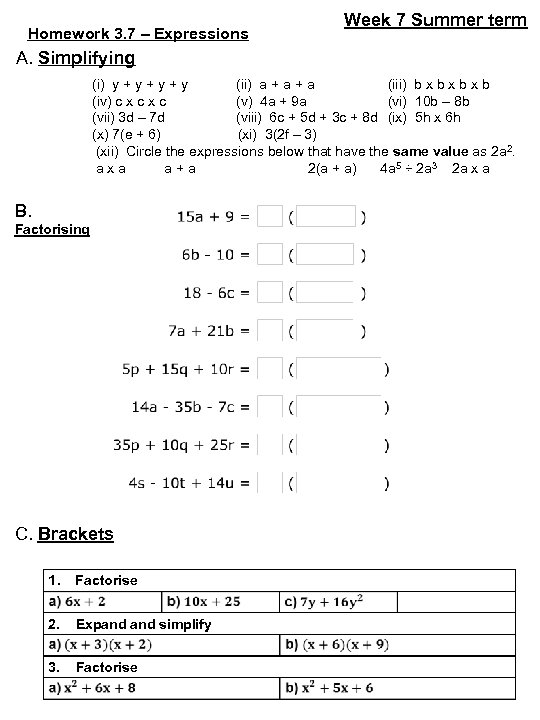Homework 3. 7 – Expressions Week 7 Summer term A. Simplifying (i) y + y + y (ii) a + a (iii) b x b x b (iv) c x c (v) 4 a + 9 a (vi) 10 b – 8 b (vii) 3 d – 7 d (viii) 6 c + 5 d + 3 c + 8 d (ix) 5 h x 6 h (x) 7(e + 6) (xi) 3(2 f – 3) (xii) Circle the expressions below that have the same value as 2 a 2. a x a a + a 2(a + a) 4 a 5 ÷ 2 a 3 2 a x a B. Factorising C. Brackets 1. Factorise 2. Expand simplify 3. Factorise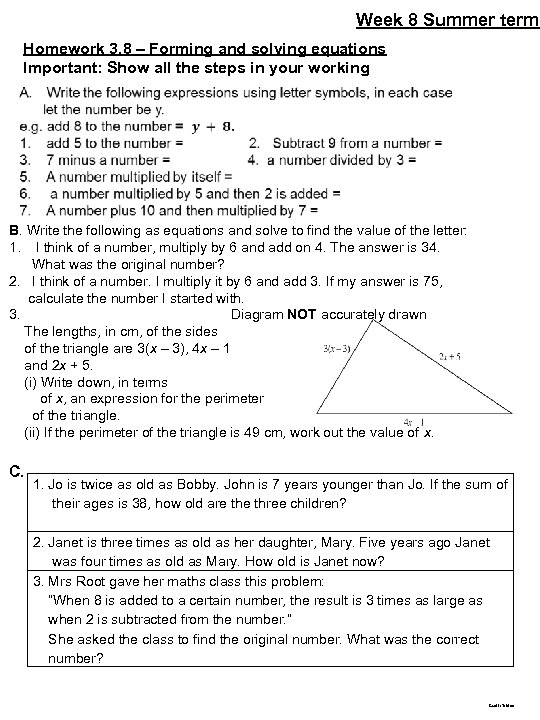Week 8 Summer term Homework 3. 8 – Forming and solving equations Important: Show all the steps in your working B. Write the following as equations and solve to find the value of the letter: 1. I think of a number, multiply by 6 and add on 4. The answer is 34. What was the original number? 2. I think of a number. I multiply it by 6 and add 3. If my answer is 75, calculate the number I started with. 3. Diagram NOT accurately drawn The lengths, in cm, of the sides of the triangle are 3(x – 3), 4 x – 1 and 2 x + 5. (i) Write down, in terms of x, an expression for the perimeter of the triangle. (ii) If the perimeter of the triangle is 49 cm, work out the value of x. C. 1. Jo is twice as old as Bobby. John is 7 years younger than Jo. If the sum of their ages is 38, how old are three children? 2. Janet is three times as old as her daughter, Mary. Five years ago Janet was four times as old as Mary. How old is Janet now? 3. Mrs Root gave her maths class this problem: “When 8 is added to a certain number, the result is 3 times as large as when 2 is subtracted from the number. ” She asked the class to find the original number. What was the correct number? Credit: Tristan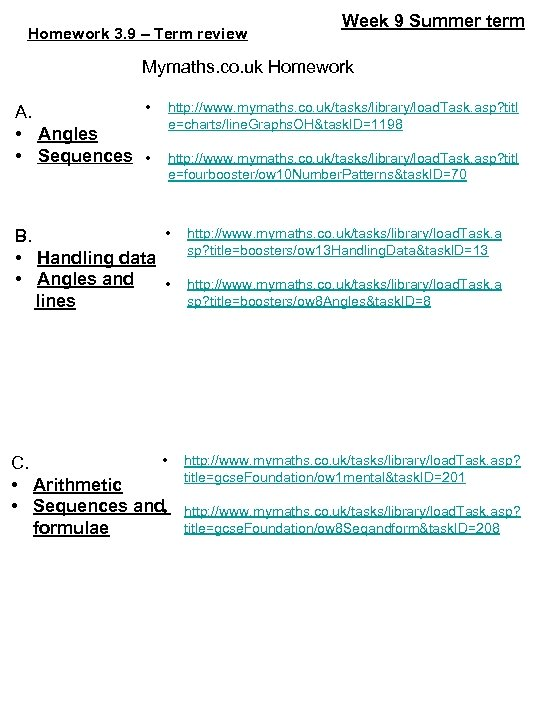Homework 3. 9 – Term review Week 9 Summer term Mymaths. co. uk Homework • Angles • Sequences • http: //www. mymaths. co. uk/tasks/library/load. Task. asp? titl e=charts/line. Graphs. OH&task. ID=1198 http: //www. mymaths. co. uk/tasks/library/load. Task. asp? titl e=fourbooster/ow 10 Number. Patterns&task. ID=70 • B. • Handling data • Angles and • lines http: //www. mymaths. co. uk/tasks/library/load. Task. a sp? title=boosters/ow 13 Handling. Data&task. ID=13 • C. • Arithmetic • Sequences and • formulae http: //www. mymaths. co. uk/tasks/library/load. Task. asp? title=gcse. Foundation/ow 1 mental&task. ID=201 http: //www. mymaths. co. uk/tasks/library/load. Task. a sp? title=boosters/ow 8 Angles&task. ID=8 http: //www. mymaths. co. uk/tasks/library/load. Task. asp? title=gcse. Foundation/ow 8 Seqandform&task. ID=208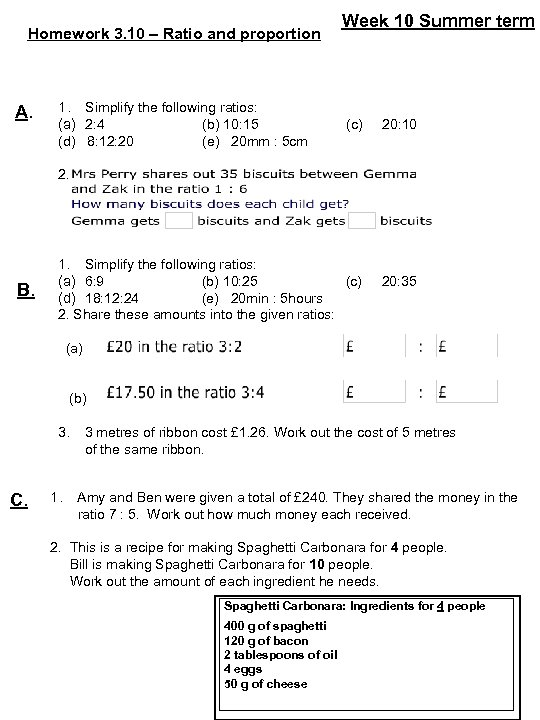Homework 3. 10 – Ratio and proportion A. 1. Simplify the following ratios: (a) 2: 4 (b) 10: 15 (d) 8: 12: 20 (e) 20 mm : 5 cm Week 10 Summer term (c) 20: 10 2. B. 1. Simplify the following ratios: (a) 6: 9 (b) 10: 25 (c) 20: 35 (d) 18: 12: 24 (e) 20 min : 5 hours 2. Share these amounts into the given ratios: (a) (b) 3. 3 metres of ribbon cost £ 1. 26. Work out the cost of 5 metres of the same ribbon. C. 1. Amy and Ben were given a total of £ 240. They shared the money in the ratio 7 : 5. Work out how much money each received. 2. This is a recipe for making Spaghetti Carbonara for 4 people. Bill is making Spaghetti Carbonara for 10 people. Work out the amount of each ingredient he needs. Spaghetti Carbonara: Ingredients for 4 people 400 g of spaghetti 120 g of bacon 2 tablespoons of oil 4 eggs 50 g of cheese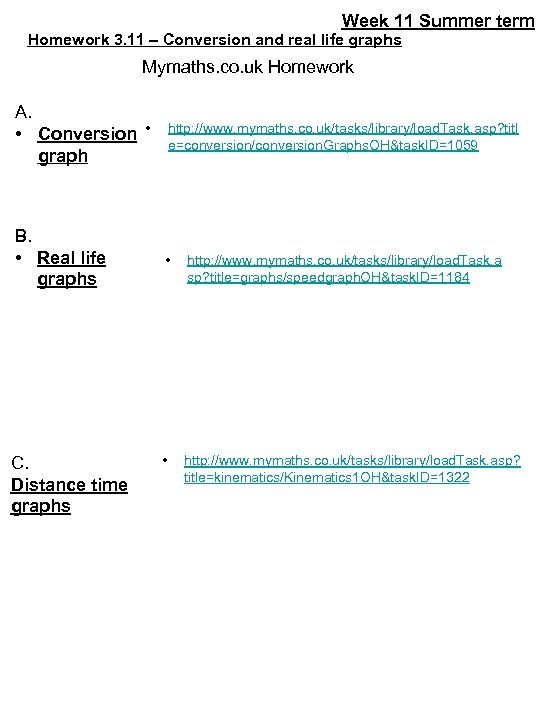Week 11 Summer term Homework 3. 11 – Conversion and real life graphs Mymaths. co. uk Homework A. • Conversion • graph http: //www. mymaths. co. uk/tasks/library/load. Task. asp? titl e=conversion/conversion. Graphs. OH&task. ID=1059 B. • Real life graphs • http: //www. mymaths. co. uk/tasks/library/load. Task. a sp? title=graphs/speedgraph. OH&task. ID=1184 • http: //www. mymaths. co. uk/tasks/library/load. Task. asp? title=kinematics/Kinematics 1 OH&task. ID=1322 C. Distance time graphs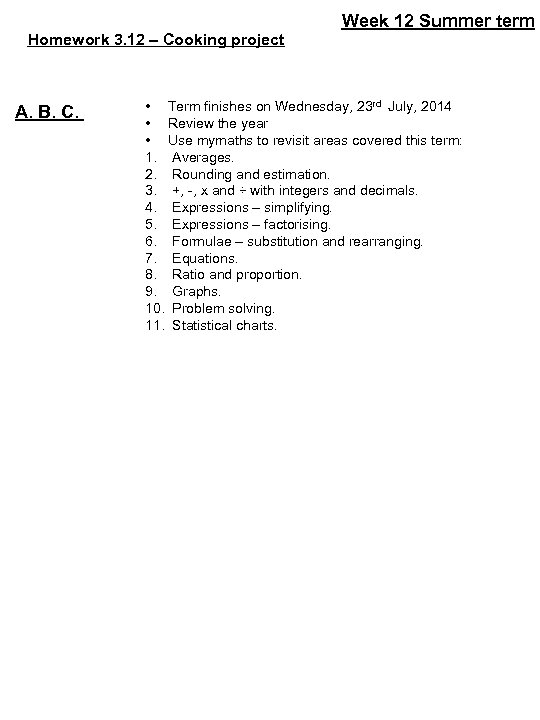Homework 3. 12 – Cooking project A. B. C. Week 12 Summer term • Term finishes on Wednesday, 23 rd July, 2014 • Review the year • Use mymaths to revisit areas covered this term: 1. Averages. 2. Rounding and estimation. 3. +, -, x and ÷ with integers and decimals. 4. Expressions – simplifying. 5. Expressions – factorising. 6. Formulae – substitution and rearranging. 7. Equations. 8. Ratio and proportion. 9. Graphs. 10. Problem solving. 11. Statistical charts.